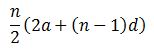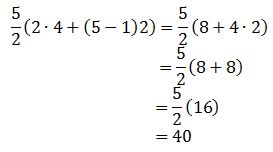# Understanding Arithmetic Series in Algebra

An error occurred trying to load this video.

Try refreshing the page, or contact customer support.

Coming up next: Working with Geometric Sequences

### You're on a roll. Keep up the good work!

Replay
Your next lesson will play in 10 seconds
• 0:01 Arithmetic Sequence
• 0:43 Arithmetic Series
• 1:15 Finding the Common Difference
• 2:23 Arithmetic Series Sum
• 5:05 Lesson Summary
Save Save

Want to watch this again later?

Timeline
Autoplay
Autoplay
Speed Speed Audio mode

#### Recommended Lessons and Courses for You

Lesson Transcript
Instructor: Yuanxin (Amy) Yang Alcocer

Amy has a master's degree in secondary education and has taught math at a public charter high school.

In this video lesson, we will learn about arithmetic series and how they work. Learn also how to find the pattern of an arithmetic sequence along with finding the sum.

## Arithmetic Sequence

Arithmetic sequences, or strings of numbers where each number is the previous number plus a constant, can be found all around us. Simply start counting and you are saying the numbers of a very famous arithmetic sequence, our numbers! It goes 1, 2, 3 and so on. Notice how each number is the previous number plus one? This difference between our numbers is called the common difference. We write an arithmetic sequence with curly brackets and commas between the numbers. So our counting numbers can be written {1, 2, 3, . . .} with the three dots in the end telling us that the sequence continues indefinitely.

## Arithmetic Series

If we add up a few or all of the numbers in our sequence, then we have what is called an arithmetic series. In other words, an arithmetic series is the sum of the numbers in our arithmetic sequence. You will come across math problems that will ask you to find the sum of an arithmetic sequence as you continue on in your math lessons. Keep watching, and I will show you a formula you can use to find this sum. But before we can do that, we need to find the common difference.

## Finding the Common Difference

Finding our common difference is an easy process. What you do is you take any pair of successive numbers and you subtract the first from the second. Now, you take another pair and subtract the first from the second to see if it also has the same difference. If you get the same difference then you have found your common difference. Each arithmetic sequence will have its own common difference.

For example, the sequence {4, 6, 8, 10, . . .} is an arithmetic sequence because it has a common difference of two, because each pair of successive numbers has a difference of two between them. We have 6 - 4 = 2. We also have 8 - 6 = 2. Ten minus eight is also two.

Look at the sequence {2, 5, 8, 11, . . .}. Does this sequence have a common difference? We subtract 5 - 2, we get 3. What is 8 minus 5? Is it also 3? Yes. Okay; so far, so good. Finally, what is 11 - 8? Oh good, it is also 3. So our common difference is 3.

## Arithmetic Series Sum

Now that we know how to find our common difference, we can go about and find our arithmetic series sum. We can use the formula n / 2 (2a (n - 1)d).The n represents the number of terms in our series that we are adding up. If we want to add the first 10 numbers, for example, then n = 10. Our problem will tell us how many numbers we are adding up. The a represents the beginning term of our sequence, and d is the common difference. So for our sequence {4, 6, 8, 10, . . .} from before, our a is 4, and our d is 2. If we want to find the sum of the first five terms, then n = 5. Let's go ahead and calculate this. We plug in all our values and evaluate to see what we get.We get an answer of 40.

To unlock this lesson you must be a Study.com Member.

### Register to view this lesson

Are you a student or a teacher?

#### See for yourself why 30 million people use Study.com

##### Become a Study.com member and start learning now.
Back
What teachers are saying about Study.com

### Earning College Credit

Did you know… We have over 200 college courses that prepare you to earn credit by exam that is accepted by over 1,500 colleges and universities. You can test out of the first two years of college and save thousands off your degree. Anyone can earn credit-by-exam regardless of age or education level.